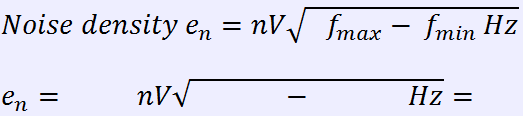Noise calculator/converter
Return back

Noise formula calculation toolkit0.0000 0.0000
This formula apply also for input-referred noise. If you need output noise estimation, it would multiply by gain.

Gain (Av) =